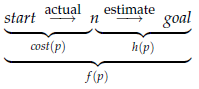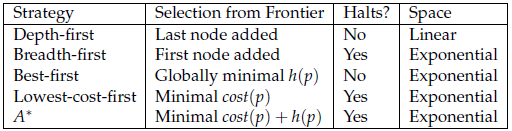A∗ Search: A∗ search is a combination of lowest-cost-first and best-first searches that considers both path cost and heuristic information in its selection of which path to expand. For each path on the frontier, A∗ uses an estimate of the total path cost from a start node to a goal node constrained to start along that path. It uses cost(p), the cost of the path found, as well as the heuristic function h(p), the estimated path cost from the end of p to the goal.

For any path p on the frontier, define f (p) = cost(p) h(p). This is an estimate of the total path cost to follow path p then go to a goal node. If n is the node at the end of path p, this can be depicted as follows:If h(n) is an underestimate of the path costs from node n to a goal node, then f (p) is an underestimate of a path cost of going from a start node to a goal node via p.

A∗ is implemented by treating the frontier as a priority queue ordered by f (p).

The property that A∗ always finds an optimal path, if one exists, and that the first path found to a goal is optimal is called the admissibility of A∗. Admissibility means that, even when the search space is infinite, if solutions exist, a solution will be found and the first path found will be an optimal solution – a lowest-cost path from a start node to a goal node.

Proposition :  (A∗ admissibility): If there is a solution, A∗ always finds a solution, and the first solution found is an optimal solution, if

• the branching factor is finite (each node has only a finite number of neighbors),
• arc costs are greater than some  > 0, and
• h(n) is a lower bound on the actual minimum cost of the lowest-cost path from n to a goal node.

Proof. Part A: A solution will be found. If the arc costs are all greater than some  > 0, eventually, for all paths p in the frontier, cost(p) will exceed any finite number and, thus, will exceed a solution cost if one exists (at depth in the search tree no greater than m/, where m is the solution cost). Because the branching factor is finite, only a finite number of nodes must be expanded before the search tree could get to this size, but the A∗ search would have found a solution by then.

Part B: The first path to a goal selected is an optimal path. The f -value for any node on an optimal solution path is less than or equal to the f -value of an optimal solution. This is because h is an underestimate of the actual cost from a node to a goal. Thus, the f -value of a node on an optimal solution path is less than the f -value for any non-optimal solution. Thus, a non-optimal solution can never be chosen while a node exists on the frontier that leads to an optimal solution (because an element with minimum f -value is chosen at each step). So, before it can select a non-optimal solution, it will have to pick all of the nodes on an optimal path, including each of the optimal solutions. It should be noted that the admissibility of A∗ does not ensure that every intermediate node selected from the frontier is on an optimal path from the start node to a goal node. Admissibility relieves the algorithm from worrying about cycles and ensures that the first solution found will be optimal. It does not ensure that the algorithm will not change its mind about which partial path is the best while it is searching.

To see how the heuristic function improves the efficiency of A∗, suppose c is the cost of a shortest path from a start node to a goal node. A∗, with an admissible heuristic, expands every path from a start node in the set

{p : cost(p) h(p) < c}

and some of the paths in the set
{p : cost(p) h(p) = c}.
Improving h affects the efficiency of A∗ if it reduces the size of the first of these sets.

Figure 3.9: Summary of search strategies“Halts?” means “Is the method guaranteed to halt if there is a path to a goal on a (possibly infinite) graph with a finite number of neighbors for each node and where the arc costs have a positive lower bound?” Those search strategies where the answer is “Yes” have worst-case time complexity which increases exponentially with the size of the path length. Those algorithms that are not guaranteed to halt have infinite worst-case time complexity. Space refers to the space complexity, which is either “Linear” in the path length or “Exponential” in the path length.